Courses

# Frequency polygon and frequency curve - Presentation of Data, Business Mathematics & Statistics B Com Notes | EduRev

## B Com : Frequency polygon and frequency curve - Presentation of Data, Business Mathematics & Statistics B Com Notes | EduRev

The document Frequency polygon and frequency curve - Presentation of Data, Business Mathematics & Statistics B Com Notes | EduRev is a part of the B Com Course Business Mathematics and Statistics.
All you need of B Com at this link: B Com

FREQUENCY POLYGON
A frequency polygon is obtained by plotting the frequency of the class against its class marks, and joining the parts with line segments.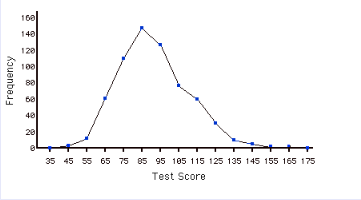Frequency polygon can also be drawn with the help of histogram by joining their mid points of rectangle.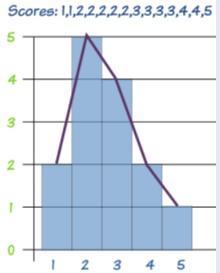Frequency Curve
When the consecutive points on the graph join with a specific pattern smoothly shown in the below example. Frequency curve can also be drawn with the help of histogram by joining their mid points of rectangle. Frequency polygon and frequency curves are same except frequency curve is drawn using free hand and frequency polygon is drawn using scale. (Each point is joint using scale)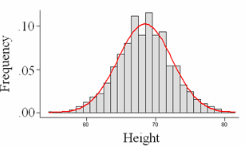CUMULATIVE FREQUENCY CURVE

The cumulative frequency curve/polygon is obtained by plotting the cumulative frequency against the upper class boundary.
In cumulative frequency curve we draw using using free hand and in cumulative frequency polygon we draw each point using scale.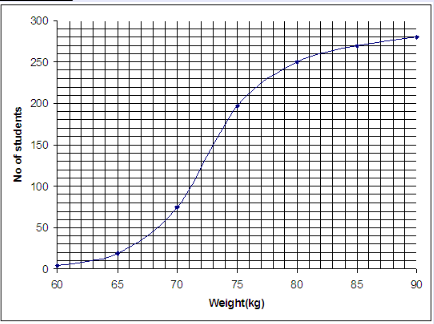To draw this graph we have to make cumulative frequency table. We add up all the frequency step by step.
For example#000000 solid;margin:15px;width:95%;">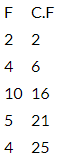After calculating CF which is cumulative frequency we plot the points and join it.

Offer running on EduRev: Apply code STAYHOME200 to get INR 200 off on our premium plan EduRev Infinity!

122 videos|142 docs

,

,

,

,

,

,

,

,

,

,

,

,

,

,

,

,

,

,

,

,

,

,

,

,

;